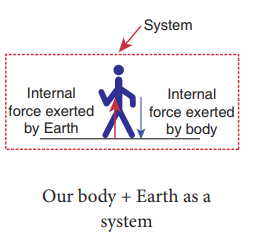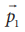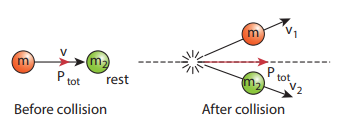Home | | Physics 11th std | Solved Example Problems on Law of Conservation of Total Linear Momentum

# Solved Example Problems on Law of Conservation of Total Linear Momentum

Identify the internal and external forces acting on the following systems. a. Earth alone as a system b. Earth and Sun as a system c. Our body as a system while walking. d. Our body + Earth as a system

Example 3.15

Identify the internal and external forces acting on the following systems.

a. Earth alone as a system

b. Earth and Sun as a system

c. Our body as a system while walking.

d. Our body + Earth as a system

## a. Earth alone as a system

Earth orbits the Sun due to gravitational attraction of the Sun. If we consider Earth as a system, then Sun’s gravitational force is an external force. If we take the Moon into account, it also exerts an external force on Earth.## b. (Earth + Sun) as a system

In this case, there are two internal forces which form an action and reaction pair-the gravitational force exerted by the Sun on Earth and gravitational force exerted by the Earth on the Sun.## c. Our body as a system

While walking, we exert a force on the Earth and Earth exerts an equal and opposite force on our body. If our body alone is considered as a system, then the force exerted by the Earth on our body is external.## d. (Our body + Earth) as a system

In this case, there are two internal forces present in the system. One is the force exerted by our body on the Earth and the other is the equal and opposite force exerted by the Earth on our body.Meaning of law of conservation of momentum

1. The Law of conservation of linear momentum is a vector law. It implies that both the magnitude and direction of total linear momentum are constant. In some cases, this total momentum can also be zero.

2. To analyse the motion of a particle, we can either use Newton’s second law or the law of conservation of linear momentum. Newton’s second law requires us to specify the forces involved in the process. This is difficult to specify in real situations. But conservation of linear momentum does not require any force involved in the process. It is covenient and hence important.

For example, when two particles collide, the forces exerted by these two particles on each other is difficult to specify. But it is easier to apply conservation of linear momentum during the collision process.Examples

Consider the firing of a gun. Here the system is Gun+bullet. Initially the gun and bullet are at rest, hence the total linear momentum of the system is zero. Letbe the momentum of the bullet andthe momentum of the gun before firing. Since initially both are at rest,Consider two particles. One is at rest and the other moves towards the first particle (which is at rest). They collide and after collison move in some arbitrary directions. In this case, before collision, the total linear momentum of the system is equal to the initial linear momentum of the moving particle. According to conservation of momentum, the total linear momentum after collision also has to be in the forward direction. The following figure explains this.A more accurate calculation is covered in section 4.4. It is to be noted that the total momentum vector before and after collison points in the same direction. This simply means that the total linear momentum is constant before and after the collision. At the time of collision, each particle exerts a force on the other. As the two particles are considered as a system, these forces are only internal, and the total linear momentum cannot be altered by internal forces.

Study Material, Lecturing Notes, Assignment, Reference, Wiki description explanation, brief detail
11th Physics : UNIT 3 : Laws of Motion : Solved Example Problems on Law of Conservation of Total Linear Momentum |